# Seeing Stars

Let us now take a closer look at the hex-fractal we sliced last week. Chopping a level 0, 1, 2, and 3 Menger sponge through our slanted plane gives the following:Studying the slicings of various Menger sponge levels gives an algorithm for describing the hex-fractal.

This suggests an iterative recipe to generate the hex-fractal. Any time we see a hexagon, chop it into six smaller hexagons and six triangles as illustrated below. Similarly, any time we see a triangle, chop it into a hexagon and three triangles like this:A recipe for constructing the hex-fractal: replace each hexagon with 6 hexagons and 6 triangles, and replace each triangle with 1 hexagon and 3 triangles.

In the limit, each triangle and hexagon in the above image becomes a hex-fractal or a tri-fractal, respectively. The final hex-fractal looks something like this (click for larger image):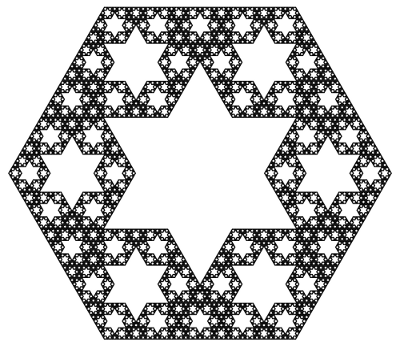Now we are in a position to answer last week’s question: how can we compute the Hausdorff dimension of the hex-fractal? Let d be its dimension. Like last week, our computation will proceed by trying to compute the “d-dimensional volume” of our shape. So, start with a “large” hex-fractal and tri-fractal, each of side-length 1, and let their d-dimensional volumes be h and t respectively.

Break these into “small” hex-fractals and tri-fractals of side-length 1/3, so these have volumes $$h/3^d$$ and $$t/3^d$$ respectively (this is how “d-dimenional stuff” scales). Since $$\begin{gather*}(\text{large hex}) = 6(\text{small hex})+6(\text{small tri}) \quad \text{and}\\ (\text{large tri}) = (\text{small hex})+3(\text{small tri}),\end{gather*}$$ we find that $$h=6h/3^d + 6t/3^d$$ and $$t=h/3^d+3t/3^d$$. Surprisingly, this is enough information to solve for the value of $$3^d$$. We find $$3^d = \frac{1}{2}(9+\sqrt{33})$$, so $$d=\log_3\left(\frac{9+\sqrt{33}}{2}\right) = 1.8184\ldots,$$ as claimed last week.

As a final thought, why did we choose to slice the Menger sponge on this plane? Why not any of the (infinitely many) others? Even if we only look at planes parallel to our chosen plane, a mesmerizing pattern emerges:

It takes a bit more work to turn the above computation of the hex-fractal’s dimension into a full proof, but there are a few ways to do it. Possible methods include mass distributions or similarity graphs.

This diagonal slice through the Menger sponge has been proposed as an exhibit at the Museum of Math. Sebastien Perez Duarte seems to have been the first to slice a Menger sponge in this way (see his rendering), and his animated cross section inspired my animation above.

### Notes

1. We’re assuming that the hex-fractal and tri-fractal have the same Hausdorff dimension. This is true, and it follows from the fact that a scaled version of each lives inside the other. []
2. There are actually two solutions, but the fact that h and t are both positive rules one out. []
3. Proposition 4.9 in: Kenneth Falconer. Fractal Geometry: Mathematical Foundations and Applications. John Wiley & Sons: New York, 1990. []
4. Section 6.6 in: Gerald Edgar. Measure, Topology, and Fractal Geometry (Second Edition). Springer: 2008. []

# A Balancing Fact

Enough geometry already! Let’s switch gears and explore an interesting phenomenon in balanced grid coloring:

Problem: The numbers 1,2,…,100 are written in order in a $$10\times 10$$ grid. Some grid cells are colored red, and the rest blue, in such a way that exactly half the cells in each row and each column are red (call this a balanced coloring). Show that the sum of the numbers in red cells equals the sum of the numbers in blue cells.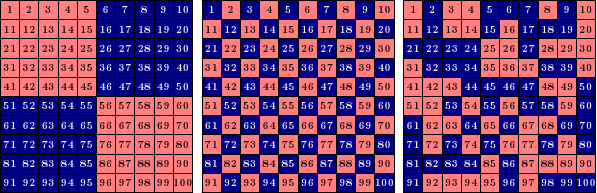Examples of balanced grids: each grid has five red and five blue cells in each row and column. By the problem above, the red numbers and blue numbers add to the same total in each grid.

How can we understand this question? Notice that each row needs five red and five blue cells, but the values in each row do not need to balance. Indeed, the first row of the first example above is very skewed toward blue: $$\text{red}=1+2+3+4+5=15$$ and $$\text{blue}=6+7+8+9+10=40$$. In fact, every row in that example is very skewed to one side or the other! But the fact that every column also needs five red and five blue numbers somehow forces these skewed row sums to cancel each other out. This is essentially what the problem asks us to prove.

### A (Not So Great) Start

Here’s one idea: let’s just test all the balanced grid colorings! There can’t be too many, right? Let’s start small. How many balanced $$2\times 2$$ grid colorings are there? (Each row needs 1 red and 1 blue.) Just two: the first cell is either red or blue, and everything else is determined from there (cf. binary sudoku). And each of these has a red sum and a blue sum of 5, so we’ve verified the problem for $$2\times 2$$ grids. What about $$4\times 4$$? With a little work, you can compute by hand that there are 90 balanced colorings of a $$4\times 4$$ grid (try this!). That’s already a lot of cases to check. For $$10\times 10$$ grids, it turns out that there are 6736218287430460752 balanced colorings! Maybe it’s time to look for a different method.

### A Solution

Here’s a method that does work. Start with a zero in each grid cell; the red and blue sums are certainly equal (they’re both zero). Now we’ll modify the values one row or column at a time. If we add 1 to each cell in a column, then the red and blue sums each increase by 5 (because each column is balanced), so the sums remain equal. Do this once in the first column, twice in the second column, and so on, resulting in the middle grid below (which must therefore have equal red and blue sums):Adding the same number to each cell in a row or column of a balanced grid keeps the red and blue sums equal. Applying this process to an initially all-zero grid yields the desired grid of 1,2,...,100 and solves the problem.

Now modify the rows: add 10 to the second row, 20 to the third row, etc., finally ending up with the numbers 1,2,…,100 in order. Each step preserves the fact that the red and blue sums match, so we’re done! Notice that this argument works for any even grid size; 10 is not special here.

This problem appeared (with different phrasing) in the 2001 Putnam Competition, a very challenging mathematics competition for undergraduate students in the United States and Canada. This competition’s directories contain even more solutions and insights into this problem (Problem 2001 B1).

# Spherical Surfaces and Hat Boxes

To round off our series on round objects (see the first and second posts), let’s compute the sphere’s surface area. We can compute this in the same way we related the area and circumference of a circle two weeks ago. Approximate the surface of the sphere with lots of small triangles, and connect these to the center of the sphere to create lots of triangular pyramids. Each pyramid has volume $$\frac{1}{3}(\text{area of base})(\text{height})$$, where the heights are all nearly $$r$$ and the base areas add to approximately the surface area. By using more and smaller triangles these approximations get better and better, so the volume of the sphere is $$\frac{4}{3}\pi r^3 = \frac{1}{3}(\text{surface area})\cdot r,$$ meaning the surface area is $$4\pi r^2$$. (This and previous arguments can be made precise with the modern language of integral calculus.)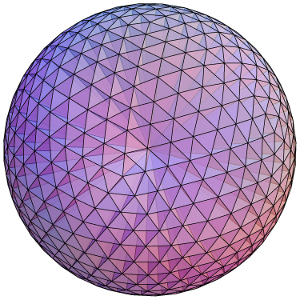Dividing a sphere into many pyramids connected to the center allows us to relate the sphere's surface area and volume.

Here’s an elegant way to rephrase this result: The surface area of a sphere is equal to the area of the curved portion of a cylinder that exactly encloses the sphere. In fact, something very surprising happens here!:

Archimedes’ Hat-Box Theorem: If we draw any two horizontal planes as shown below, then the portions of the sphere and the cylinder between the two planes have the same surface area.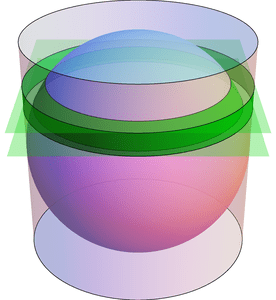Any two horizontal planes cut off a band on the sphere and another band on the enclosing cylinder. Archimedes' Hat-Box Theorem says that these bands have the same area. (The planes shown here have heights $$0.4\cdot r$$ and $$0.6\cdot r$$ above the equator.)

We can prove this with (all!) the methods in the last few posts; here’s a quick sketch. To compute the area of the “spherical band” (usually called a spherical zone), first consider the solid spherical sector formed by joining the spherical zone to the center: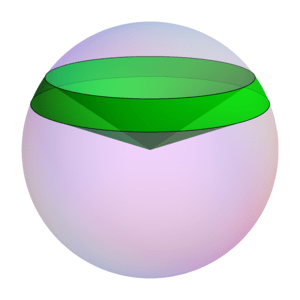The Hat-Box theorem can be proved by relating the area of the spherical zone to the volume of this spherical sector.

By dividing this into lots of triangular pyramids as we did with the sphere above, we can compute the area of the spherical zone by instead computing the sector’s volume. This volume can be computed by breaking it into three parts: two cones and the spherical segment between the two planes (on the left of the next figure). Compute the volume of the spherical segment by comparing (via Cavalieri’s Principle) to the corresponding part of the vase (from the previous post), which can be expressed with just cylinders and cones.Computing the volume of a spherical segment via Cavalieri's Principle

See if you can fill in the details!

# Slicing Spheres

Last week we saw how to compute the area of a circle from first principles. What about spheres?

To compute the volume of a sphere, let’s show that a hemisphere (with radius $$r$$) has the same volume as the vase shown in the figure below, formed by carving a cone from the circular cylinder with radius and height $$r$$. Why this shape? Here’s why: if we cut these two solids at any height $$h$$ (between 0 and $$r$$), the areas of the two slices match. Indeed, the slice—usually called cross section—of the sphere is a circle of radius $$\sqrt{r^2-h^2}$$, which has area $$\pi(r^2-h^2)$$. Similarly, the vase’s cross section is a radius $$r$$ circle with a radius $$h$$ circle cut out, so its area is $$\pi r^2-\pi h^2$$, as claimed.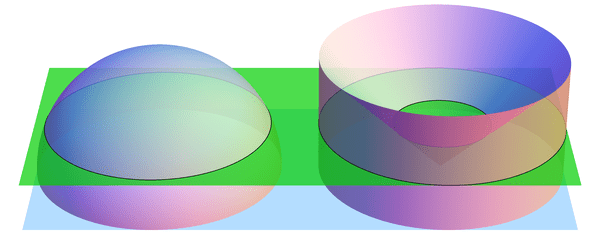When sliced by a horizontal plane at any height $$h$$, the hemisphere and vase have equal cross-sectional areas. (Shown here for $$h = 0.4\cdot r$$.) By Cavalieri's Principle, this implies that they have equal volumes.

If we imagine the hemisphere and vase as being made from lots of tiny grains of sand, then we just showed, intuitively, that the two solids have the same number of grains of sand in every layer. So there should be the same number of grains in total, i.e., the volumes should match. This intuition is exactly right:

Cavalieri’s Principle: any two shapes that have matching horizontal cross sectional areas also have the same volume.

So the volumes are indeed equal, and all that’s left is to compute the volume of the vase. But we can do this! Recall that the cone has volume $$\frac{1}{3} (\text{area of base}) (\text{height}) = \frac{1}{3}\pi r^3$$ (better yet, prove this too! Hint: use Cavalieri’s Principle again to compare to a triangular pyramid). Likewise, the cylinder has volume $$(\text{area of base}) (\text{height}) = \pi r^3$$, so the vase (and hemisphere) have volume $$\pi r^3 – \frac{1}{3} \pi r^3 = \frac{2}{3}\pi r^3$$. The volume of the whole sphere is thus $$\frac{4}{3}\pi r^3$$. Success!

The following visualization illustrates what we have shown, namely $$\text{hemisphere} + \text{cone} = \text{cylinder}.$$ The “grains of sand” in the hemisphere are being displaced horizontally by the stabbing cone, and at the end we have exactly filled the cylinder.Stabbing a cone into a hemisphere made of horizontally-moving "sand particles" exactly fills a cylinder.

# Archimedes’ Circular Reasoning

Every geometry textbook has formulas for the circumference ($$C = 2 \pi r$$) and area ($$A = \pi r^2$$) of a circle. But where do these come from? How can we prove them?

Well, the first is more a definition than a theorem: the number $$\pi$$ is usually defined as the ratio of a circle’s circumference to its diameter: $$\pi = C/(2r)$$. Armed with this, we can compute the area of a circle. Archimedes’ idea (in 260 BCE) was to approximate this area by looking at regular $$n$$-sided polygons drawn inside and outside the circle, as in the diagram below. Increasing $$n$$ gives better and better approximations to the area.The n-sided regular polygons inside (blue) and outside (red) a circle can be used to approximate the area of the circle. Shown here for n=12.

Look first at the inner polygon. Its perimeter is slightly less than the circle’s circumference, $$C = 2 \pi r$$, and the height of each triangle is slightly less than $$r$$. So when reassembled as shown, the triangles form a rectangle whose area is just under $$C/2\cdot r = \pi r^2$$. Likewise, the outer polygon has area just larger than $$\pi r^2$$. As $$n$$ gets larger, these two bounds get closer and closer to $$\pi r^2$$, which is therefore the circle’s area.

Archimedes used this same idea to approximate the number $$\pi$$. Not only was he working by hand, but the notion of “square root” was not yet understood well enough to compute with. Nevertheless, he was amazingly able to use 96-sided polygons to approximate the circle! His computation included impressive dexterity with fractions: for example, instead of being able to use $$\sqrt{3}$$ directly, he had to use the (very close!) approximation $$\sqrt{3} > 265/153$$. In the end, he obtained the bounds $$3\frac{10}{71} < \pi < 3\frac{1}{7}$$, which are accurate to within 0.0013, or about .04%. (In fact, he proved the slightly stronger but uglier bounds $$3\frac{1137}{8069} < \pi < 3\frac{1335}{9347}$$. See this translation and exposition for more information on Archimedes’ methods.)

These ideas can be pushed further. Focus on a circle with radius 1. The area of the regular $$n$$-sided polygon inscribed in this circle can be used as an approximation for the circle’s area, namely $$\pi$$. This polygon has area $$A_n = n/2 \cdot \sin(360/n)$$ (prove this!). What happens when we double the number of sides? The approximation changes by a factor of $$\frac{A_{2n}}{A_n} = \frac{2\sin(180/n)}{\sin(360/n)} = \frac{1}{\cos(180/n)}.$$ Starting from $$A_4 = 2$$, we can use the above formula to compute $$A_8,A_{16},A_{32},\ldots$$, and in the limit we find that $$\pi = \frac{2}{\cos(180/4)\cdot\cos(180/8)\cdot\cos(180/16)\cdots}.$$ Finally, recalling that $$\cos(180/4) = \cos(45) = \sqrt{\frac{1}{2}}$$ and $$\cos(\theta/2) = \sqrt{\frac{1}{2}(1+\cos\theta)}$$ (whenever $$\cos(\theta/2) \ge 0$$), we can rearrange this into the fun infinite product $$\frac{2}{\pi} = \sqrt{\frac{1}{2}} \cdot \sqrt{\frac{1}{2}+\frac{1}{2}\sqrt{\frac{1}{2}}} \cdot \sqrt{\frac{1}{2}+\frac{1}{2}\sqrt{\frac{1}{2}+\frac{1}{2}\sqrt{\frac{1}{2}}}} \cdots$$ (which I found at Mathworld). (It’s ironic that this formula for a circle uses so many square roots!)

# A Distinctly Odd Coincidence

This week’s topic comes from partition theory. Consider all the ways to write 7 as the sum of positive integers:
\begin{align*} &7, && 3+2+2,\\ &6+1, && 3+2+1+1,\\ &5+2, && 3+1+1+1+1,\\ &5+1+1, && 2+2+2+1,\\ &4+3, && 2+2+1+1+1,\\ &4+2+1, && 2+1+1+1+1+1,\\ &4+1+1+1, && 1+1+1+1+1+1+1+1.\\ &3+3+1, && \end{align*} (We don’t care about order: for example, $$5+2$$ and $$2+5$$ are the same.) These are called the partitions of 7, and there are 15 of them.

We can also consider imposing constraints on these partitions: For example, how many of these partitions of 7 use only odd integers? These “odd” partitions are
\begin{align*} & 7, && 3+1+1+1+1, \\ & 5+1+1, && 1+1+1+1+1+1+1, \\ & 3+3+1, && \end{align*} and there are 5 of them. Here’s a very different question: how many partition of 7 have no repeated parts? These “distinct” partition are
\begin{align*} & 7, && 6+1, && 5+2, && 4+3, && 4+2+1, \end{align*} and there are 5 of these as well.

This must be coincidence, right? Let’s try a bigger example. How many partitions of 11 use only odd parts? You can (and should!) check that there are 12. And how many partitions of 11 use only distinct parts? Again, there are 12. Surprisingly, these numbers will always match!:

Theorem (Euler): For any positive integer $$n$$, the number of “odd” partitions of $$n$$ is the same as the number of “distinct” partitions of $$n$$.

### Transforming Between “Odd” and “Distinct” Partitions

Let’s try to make sense of this. Given a partition of $$n$$ into odd parts, there is a simple way to transform it into a partition with distinct parts: whenever two parts are the same, add them together, and repeat until they are all distinct. For example, the partition $$7+3+3+3+3+3+3+1+1+1$$ of 28 is transformed into
\begin{align*} & 7+(3+3)+(3+3)+(3+3)+(1+1)+1 \\ & \quad \to 7+(6+6)+6+2+1 \\ & \quad \to 12+7+6+2+1, \end{align*} which has distinct parts. In the other direction, to transform a “distinct” partition to an “odd” one, we can reverse this process: whenever there is an even part, cut it in half and write it twice. Our example $$12+7+6+2+1$$ from above becomes
\begin{align*} & (6+6)+7+(3+3)+(1+1)+1 \\ &\quad \to (3+3)+(3+3)+7+3+3+1+1+1, \end{align*} which is the “odd” permutation we started with. These transformations can be shown to match “odd” permutations with “distinct” permutations in pairs, proving that these sets have the same size. (A careful argument uses binary representation.) For example, the 5 partitions of 7 above are paired as follows:
\begin{align*} & 7 &&\leftrightarrow && 7 \\ & 6+1 &&\leftrightarrow && 3+3+1 \\ & 5+2 &&\leftrightarrow && 5+1+1 \\ & 4+3 &&\leftrightarrow && 3+1+1+1+1 \\ & 4+2+1 &&\leftrightarrow && 1+1+1+1+1+1+1. \end{align*} (If you know something about generating functions, then a different proof follows by proving the identity
$$\frac{1}{(1-x)(1-x^3)(1-x^5)(1-x^7)\cdots} = (1+x)(1+x^2)(1+x^3)(1+x^4)\cdots$$ and noting that the coefficients of $$x^n$$ in the expansions of the left and right products equals the number of “odd” and “distinct” permutations of $$n$$, respectively.)

### More Coincidences

These apparent coincidences are characteristic of many results in partition theory. Try your hand at proving the following generalization:

Theorem: The number of partitions of $$n$$ into parts that are not divisible by 10 equals the number of partitions of $$n$$ where no part is repeated 10 or more times. The same is true for any positive integer in place of 10.

(Hint: both methods used above can be extended to prove this.) If instead we want the parts to be really distinct and make sure no two parts are equal or consecutive, then we want my favorite partition theory result:

Theorem (Rogers-Ramanujan Identity): The number of partitions of $$n$$ where any two parts differ by at least 2 is the same as the number of partitions of $$n$$ where every part is one more or one less than a multiple of 5.

Despite the simplicity of the statement, this is much harder to prove than the previous theorems.

# Secret Santa (Now with Fewer Hats!)

As we approach the holiday season, some of you may soon participate in—or perhaps even organize—a “Secret Santa” exchange. Specifically, some number of people enter the exchange, and each is assigned one person (their Santee) to give a gift to (or multiple gifts… or other craziness…), in such a way that each person also receives from one person (their Santa). As organizers of such an exchange, how should we choose a permutation of the participants, i.e., an assignment of each person to his/her Santee? Here’s one method: put all names in a hat, and let each player extract a name at random. What could go wrong?

For starters, someone might pick their own name! Shopping for yourself might make the task easier, but this seems to contradict the (Christmas) spirit of the exchange. If we pick randomly as above, and if there are $$n$$ people in the exchange, what is the probability that no one picks him/herself? For large $$n$$, this answer tends toward $$1/e = .3679\ldots$$, where $$e = 2.718\ldots$$ is Euler’s number. So, with more than a 63 percent chance, someone is left out. (See below for a proof of this fact.)

What else might go wrong? Perhaps we also want to avoid two people being assigned each other, so that this pair doesn’t feel excluded. What is the chance of a randomly chosen permutation having no excluded person or pair? This can be computed to tend toward $$1/e^{3/2} = .2231\ldots$$, so there is some left out person or pair with almost 80% probability. And if we wish to make sure there are no excluded groups of size at most 6, say, then the chance of failure limits to $$1-e^{-1-\frac{1}{2}-\frac{1}{3}-\frac{1}{4}-\frac{1}{5}-\frac{1}{6}} = .9137$$ for large $$n$$.

So, while Santa hats may be fluffy and festive, Secret Santa hats are a bad idea. What should we do to organize our exchange? Here’s one option: just make a big cycle. Specifically, write all names in some random order and chain them up, e.g., $$2\to 6\to 7\to 3\to 1\to 4\to 5\to 2$$ (if the “names” are just 1, 2, …, 7).

### Where do these numbers come from?

How can we compute the probabilities mentioned above? One common method to prove the $$1/e$$ probability uses the principle of Inclusion-Exclusion, but here is a sketch of an argument that doesn’t rely on this technique. Let $$A_n$$ be the number of permutations of $$n$$ people with no person assigned to him/herself. Such a permutation is called a Derangement, and a direct counting argument can be used to show that $$A_n = (n-1)(A_{n-1} + A_{n-2})$$ (see the Wikipedia page for derangements, for example). As there are $$n!$$ total permutations of the players, the desired probability is $$P_n = A_n/n!$$, so the above formula can be manipulated to $$P_n-P_{n-1} = -\frac{1}{n}(P_{n-1}-P_{n-2})$$. This produces $$P_n-P_{n-1} = \frac{(-1)^n}{n!}$$ by induction, so we obtain $$P_n = 1-\frac{1}{1!}+\frac{1}{2!}-\frac{1}{3!}+\cdots+\frac{(-1)^n}{n!}.$$ As $$n$$ gets big this value tends toward the infinite sum $$\sum_{i=0}^\infty \frac{(-1)^i}{i!} = 1/e$$.

The other probabilities mentioned take more work. A well-suited tool is that of (Exponential) Generating Functions, which in this case help organize a more complicated Inclusion-Exclusion argument. Using these, it can be shown that the probability that a random permutation of $$n$$ players has no groups of size 6 or less is equal to the coefficient of $$x^n$$ in the power series expansion of $$\frac{1}{1-x}\cdot e^{-\left(x+\frac{x^2}{2} + \cdots + \frac{x^6}{6}\right)}.$$

# Some Strange Sums

If someone told you to compute the value of the infinite sum $$Z = 1+2+3+4+\cdots$$, you would tell them that it simply does not converge. But what if they insisted that you assign a finite value to this sum? Which value would you give? Is there any way to make sense of this question? Yes, and the answer is $$-1/12$$.

Before tackling this sum, let’s try a slightly easier one, known as Grandi’s Series: $$G = 1-1+1-1+\cdots$$. How can we compute its value? Let’s write \begin{align*}G &= 1-1+1-1+1-\cdots \text{ and}\\ G &= 0+1-1+1-1+\ldots,\end{align*} and adding these term by term gives $$2G = 1+0+0+0+0+\cdots = 1$$, i.e., $$G = 1/2$$. So we got a value! The same “trick” lets us sum $$H = 1-2+3-4+\cdots$$ like this: \begin{align*}2H &= 1 + (-2+1) + (3-2) + (-4+3) + \cdots\\ &= 1-1+1-1+\cdots\\ &= G = 1/2,\end{align*} so $$H = 1/4$$.

What’s really going on here? A good way to understand it is to look at the functions $$g(x) = 1-x+x^2-x^3+\cdots$$ and $$h(x) = 1-2x+3x^2-4x^3+\cdots$$, and to realize that we’re asking for $$g(1)$$ and $$h(1)$$, respectively. The sum defining $$g(x)$$ converges for $$-1 < x < 1[/latex], and since $g(x) + x \cdot g(x) = 1$ (this is just the "trick" from above!), we find $g(x) = 1/(1+x)$. So, even though the sum defining $g(1) = 1-1+1-\cdots$ does not converge, the limit as $x$ approaches 1 from below is well defined and equals $1/(1+1) = 1/2$. Likewise, we find that $h(x) = 1/(1+x)^2$ for $-1 < x < 1$, and the limit as $x$ goes to 1 is 1/4. This method of putting the terms as the coefficients of a power series and extrapolating with limits is called Abel Regularization. Notice, however, that this method does not work for the sum [latex]Z$$, because $$1 + 2x + 3x^2 + 4x^3 + \cdots = 1/(1-x)^2$$, and the limit as $$x$$ approaches 1 is still infinite.

A different method of summing divergent series is called Zeta Function Regularization: to compute $$a_1 + a_2 + a_3 + \cdots$$, define the function $$a_1^{-s} + a_2^{-s} + a_3^{-s} + \cdots$$ (which hopefully converges for large enough s), and try to “extend” this to a function we can evaluate at $$s=-1$$. For example, for $$H = 1-2+3-\cdots$$, the corresponding function $$1/1^2-1/2^s+1/3^s-\cdots$$ is called the Dirichlet Eta Function, $$\eta(s)$$. Analysis based on our “trick” from above (iterated infinitely many times! [producing the so-called Euler Transform]) can be used to show that $$\eta(s)$$ can be defined for all values $$s$$ (even complex values!) and that $$\eta(-1) = 1/4$$, which agrees with our computation of $$1-2+3-4+\cdots$$ above. The corresponding function for the sum $$Z$$ is called the Riemann Zeta Function, $$\zeta(s)$$, and we can compute (for all $$s$$ where this makes sense): $$\begin{gather*} \zeta(s) = \frac{1}{1^s} + \frac{1}{2^s} + \frac{1}{3^s} + \frac{1}{4^s} + \cdots,\\ 2^{-s} \zeta(s) = \frac{1}{2^s} + \frac{1}{4^s} + \frac{1}{6^s} + \frac{1}{8^s} + \cdots, \text{ and so}\\ \zeta(s)-2\cdot 2^{-s} \zeta(s) = \frac{1}{1^s}-\frac{1}{2^s}+\frac{1}{3^s}-\frac{1}{4^s}+\cdots = \eta(s).\end{gather*}$$ So $$(1-2^{1-s}) \zeta(s)$$ equals $$\eta(s)$$ everywhere (by general complex analysis facts). At $$s = -1$$, this says $$\zeta(-1) = \eta(-1)/(-3) = -1/12$$, as claimed.

# Generating Primes with Formulas

Prime numbers—namely, positive integers with no divisors other than 1 and themselves—have long fascinated mathematicians. As a result, there have been many attempts at formulas to generate prime numbers. For example, the polynomial $$x^2-x+41$$ spits out a prime number for $$x = 0, 1, 2, …, 40$$. But for $$x = 41$$, the value is $$41^2$$, which is not prime. Can we find some nonconstant polynomial $$f(x)$$ with integer coefficients that only spits out prime values? (The constant polynomial $$f(x) = 2$$ always returns a prime, but this is not very interesting.) In a word: No. There is no such polynomial. To prove this, assume $$f$$ is such a polynomial. Then $$f(0) = p$$ for some prime $$p$$, and all of the numbers $$\{f(p), f(2p), f(3p), \ldots \}$$ are divisible by $$p$$. This sequence grows toward infinity, so one of them is greater than $$p$$. Since this number has a factor of $$p$$, it is not prime.

What if we switch from polynomials to exponents? Maybe the function $$2^m + 1$$ will give us lots of primes. It can be shown that if $$2^m + 1$$ is prime then $$m$$ itself has to be a power of 2. (Indeed, if a prime $$p > 2$$ divides $$m$$, check that $$2^{m/p} + 1$$ divides $$2^m + 1$$.) So the only possibilities are the numbers $$F_n = 2^{2^n} + 1$$, which are called Fermat numbers. The first few are \begin{align*} F_0 &= 3,\\ F_1 &= 5,\\ F_2 &= 17,\\ F_3 &= 257,\\ F_4 &= 65537. \end{align*} It was long believed that all Fermat numbers were prime (Fermat himself conjectured this!), but in fact the very next one, $$F_5 = 4294967297 = 641 \cdot 6700417$$, is composite. (In Fermat’s defense, this is a large number to factor by hand!) Are there at least infinitely many Fermat primes? We currently don’t know. In fact, the Fermat primes shown above are the only known Fermat primes!

Similarly, numbers of the form $$2^n-1$$ are called Mersenne numbers. In order for this to be prime, it is necessary for $$n$$ itself to be prime. (Indeed, if $$a$$ divides $$n$$ then $$2^a-1$$ divides $$2^n-1$$.) So is $$2^p-1$$ prime for every prime $$p$$? Unfortunately, no: $$2^{11}-1$$ is not prime. But there seem to be many Mersenne primes. Thanks to the “Great Internet Mersenne Prime Search,” a.k.a. GIMPS, there are 47 Mersenne primes known, the largest of which is $$2^{43112609}-1$$, which has 12978189 digits (in base 10).

OK, so the above “prime-generating” functions don’t work very well. Here’s one that does work. It can be shown that there is some polynomial $$Z(a, b, c, d, e, f, g, h, i, j)$$ with 10 variables and integer coefficients such that any positive output from $$Z$$ (when given integer inputs) is prime, and furthermore, every prime is obtained in this way. (Note: $$Z$$ spits out many non-positive values as well.) Unfortunately, this polynomial $$Z$$ is not very practical. For one thing, its degree is about $$38!$$. (Yes, I mean 38 factorial.)